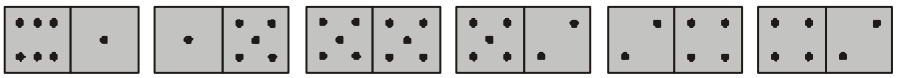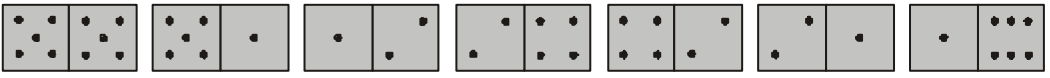시간 제한 메모리 제한 제출 정답 맞은 사람 정답 비율
1 초 128 MB 0 0 0 0.000%

## 문제

Dominoes

game played with small, rectangular blocks of wood or other material, each identified by a number of dots, or pips, on its face. The blocks usually are called bones, dominoes, or pieces and sometimes men, stones, or even cards. The face of each piece is divided, by a line or ridge, into two squares, each of which is marked as would be a pair of dice... The principle in nearly all modern dominoes games is to match one end of a piece to another that is identically or reciprocally numbered.

ENCYCLOPÆDIA BRITANNICA

Consider an arbitrary set of domino pieces where each piece is marked with two digits from 1 to 6. Some sets can be completely laid out in a row matching one end of a piece to another that is identically numbered, while others cannot. For example, the set consisting of 5 pieces: (1, 5), (1, 6), (5, 5) and (2, 4) twice, cannot be laid out in a row. However, if we add (2, 5) piece to the above set we could lay out the resulting set in the following row:However, we are interested in a row having the smallest sum of digits on its pieces. In our example, instead of the piece (2, 5) with a sum of 7, we could add two pieces (1, 2) with a total sum of 6 to lay out the following row:Your task is to write a program that for a given domino set will find an additional (possibly empty) set with the smallest possible sum of digits, so that a row could be laid out with both sets combined.

## 입력

The first line of the input file contains a single integer N (2 ≤ N ≤ 100) representing the total number of pieces in the domino set. The following N lines describe pieces. Each piece is represented on a separate line in a form of two digits from 1 to 6 separated by a space. The digits of a piece can be written in any order.

## 출력

On the first line of the output file write the smallest sum of digits of the additional set or 0 if that set is empty. On the second line write the total number of pieces in the additional set or 0 if that set is empty. Then write the pieces of the additional set in the same format as in input.

If there are a number of additional sets with the same smallest sum of digits exist then write any one of them.

## 예제 입력 1

6
6 1
1 5
5 5
5 2
2 4
4 2


## 예제 출력 1

0
0


## 예제 입력 2

5
1 5
6 1
5 5
2 4
2 4


## 예제 출력 2

6
2
1 2
1 2